## Example Questions

### Example Question #172 : Act Math

Two similiar triangles have a ratio of perimeters of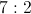.

If the smaller triangle has sides of 3, 7, and 5, what is the perimeter of the larger triangle.

Possible Answers: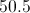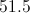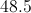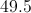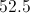Correct answer:Explanation:

Adding the sides gives a perimeter of 15 for the smaller triangle. Multipying by the given ratio of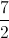, yields 52.5.

### Example Question #171 : Act Math

Two similiar triangles exist where the ratio of perimeters is 4:5 for the smaller to the larger triangle. If the larger triangle has sides of 6, 7, and 12 inches, what is the perimeter, in inches, of the smaller triangle?

Possible Answers:

20

18

23

25

Correct answer:

20

Explanation:

The larger triangle has a perimeter of 25 inches. Therefore, using a 4:5 ratio, the smaller triangle's perimeter will be 20 inches.

### Example Question #174 : Act Math

Two similar triangles' perimeters are in a ratio of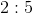. If the lengths of the larger triangle's sides are,, and, what is the perimeter of the smaller triangle?

Possible Answers: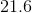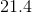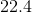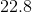Correct answer:Explanation:

1. Find the perimeter of the larger triangle: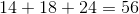2. Use the given ratio to find the perimeter of the smaller triangle: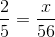Cross multiply and solve: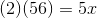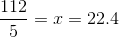### Example Question #175 : Act Math

There are two similar triangles. Their perimeters are in a ratio of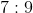. If the perimeter of the smaller triangle is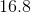, what is the perimeter of the larger triangle?

Possible Answers: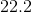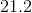Correct answer:Explanation:

Use proportions to solve for the perimeter of the larger triangle: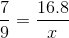Cross multiply and solve: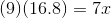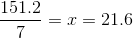### Example Question #176 : Act Math

Two similar triangles have perimeteres in the ratio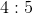. The sides of the smaller triangle measure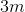,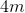, and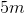respectively. What is the perimeter, in meters, of the larger triangle?

Possible Answers:Correct answer:Explanation:

Since the perimeter of the smaller triangle is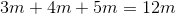, and since the larger triangle has a perimeter in theratio, we can set up the following identity, where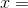the perimeter of the larger triangle: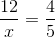In cross multiplying this identity, we get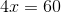. We can now solve for. Here,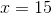, so the perimeter of the larger triangle is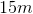.

### All ACT Math Resources